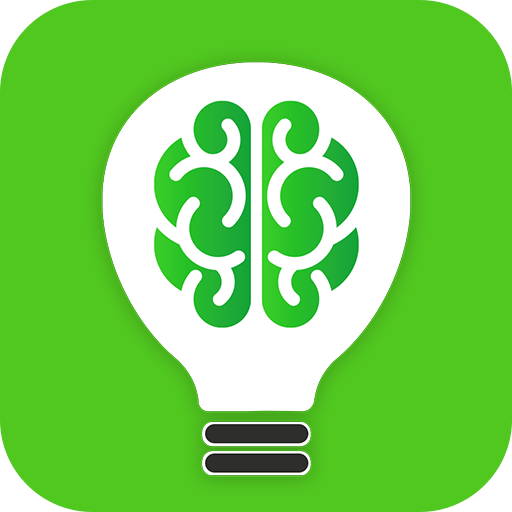# Ultimate Math Quiz App - Brain Challenge on PC/Mac

Author: AzmiSoft Inc.

Score: 0.0

OS: Windows, OS X, 4.4 +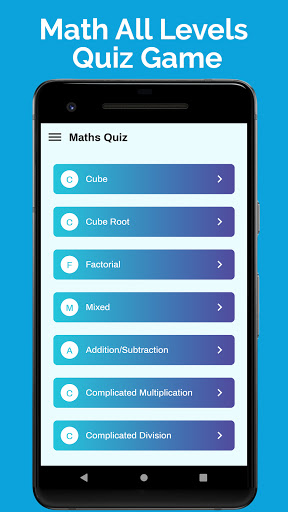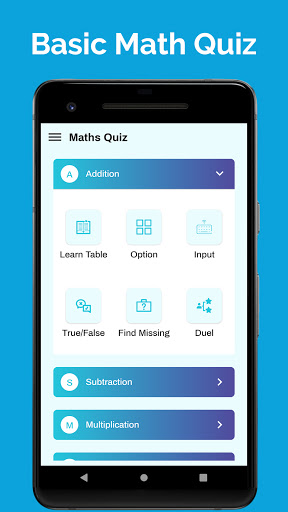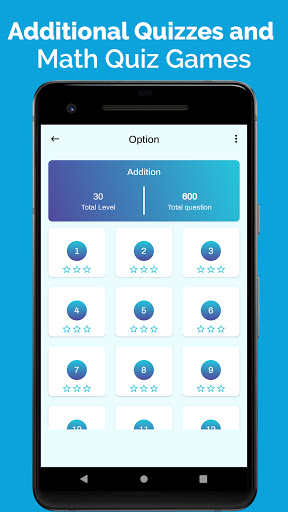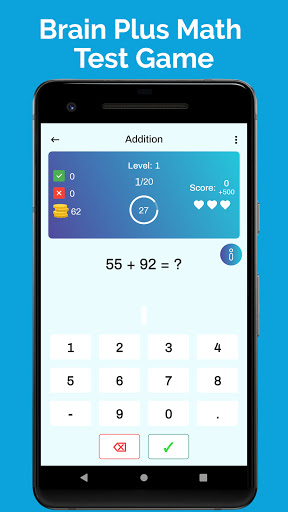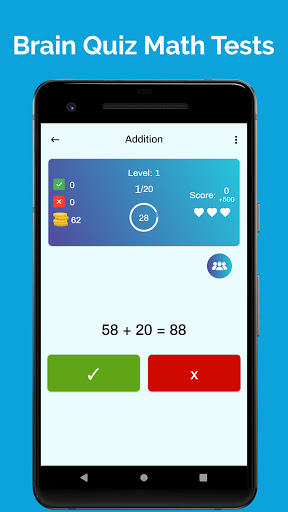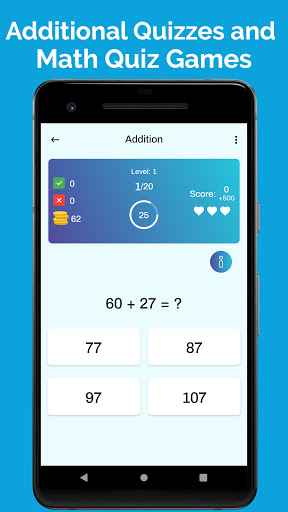##### About Ultimate Math Quiz App - Brain Challenge
Are you looking for Math All Levels Quiz Game or Fun Math Trivia Game?
Do you want to download Math Quiz Games and Brain Plus Math Test Game?

You just got the perfect Brain Quiz Math Tests named Ultimate Math Quiz App - Brain Challenge where you Additional Quizzes and Puzzles to practicing from Basic Math Quiz to Advance Math Quiz to increase your stamina of thinking and solving math problems.

Introduction to Ultimate Math Quiz App: Math Puzzle Game Brain Booster & Math All Levels Quiz Game
First of all, we warmly welcome you to our Ultimate Math Quiz App - Brain Challenge where you practice math, train your brain, and increase your thinking and problem-solving stamina. This Math Puzzle Game Brain Booster is for every age of teenagers and adults. There are different Additional Quizzes and Basic Math Quiz Games useful to practice math and speed up your calculation skills for your upcoming competitive exam. This is a type of Brain Plus Math Test Game which is a type of brain booster to give your brain a workout!

Do you love solving math problems, basic math quizzes, advance math quizzes, etc.? This is a Fun Math Trivia Game containing Brainy Math Puzzles & Quizzes which helps you to learn basic problems, equations, sequences, series, etc.

Features of this Ultimate Math Quiz App: Math Puzzle Game Brain Booster & Math All Levels Quiz Game
✪ Ultimate Math Quiz App is a 100% Free Brain Challenge Game
✪ Math Puzzle Game Brain Booster is a FREE Math All Levels Quiz Game
✪ This Brainy Math Puzzles & Quizzes also lets you set reminder with clock setting
✪ You can beat everyone by practicing in this Fun Math Trivia App contains Additional Quizzes
✪ Dual Mode Player – Turn on Competition Mode to take more interest in all Brain Quiz Math Tests
✪ There are unique basic math quizzes, and advance math quizzes in this Brain Plus Math Test Game
✪ Find out Mathematics Tips and Tricks in this Additional Quizzes and Math Quiz Games to solve problem quickly
✪ Use Brain Trivia (Math Quiz Game) on daily basis, increase your stamina, knowledge, and problem-solving stamina

Some EXTRA Features of Ultimate Math Quiz App: Math All Levels Quiz Game
✪ Math Quiz Games have Six different Themes
✪ Brain Plus Math Test Game also have option of Day, Night Mode
✪ Math All Levels Quiz Game share the result screen instead of your score
✪ All the quizzes of this Math Puzzle Game Brain Booster Game come from random logic
✪ Ultimate Math Quiz App - Brain Challenge is 100% FREE to use, practice math, and enjoy!

Ultimate Math Quiz App - Brain Challenge is a Multilingual App
This Brainy Math Puzzles & Quizzes Game supports multiple languages i.e., English, Spanish, Arabic, Hindi, Malay, Marathi, Gujrati

Some Practicing Terms Basic Math Quiz and Advance Math Quiz Game
Learn and Practice Tables and Boost your Brain with Addition, Subtraction, Multiplication, Division, Integers, Decimals, Fractional, Mixed Operators, Square, Square Root, Percentage, Cube, Cube Root, Complicated Multiplication, Complicated Division, Operation on two and three arguments, Find Missing Square, Mixed Operators, and Much More!

So, what are you waiting for? Download this Ultimate Math Quiz App: Math Puzzle Game Brain Booster & Math All Levels Quiz Game now and enjoy practicing math for FREE!

Sharing is Caring! Do you have friends who love to practice math but don’t know any simple way of practicing?
Share this Brain Plus Math Test Game: Fun Math Trivia - Additional Quizzes and Math Quiz Games with your friends and family members with your friends and family members and let them become the fastest math problem-solving person of your batch/family.
• bug fixes